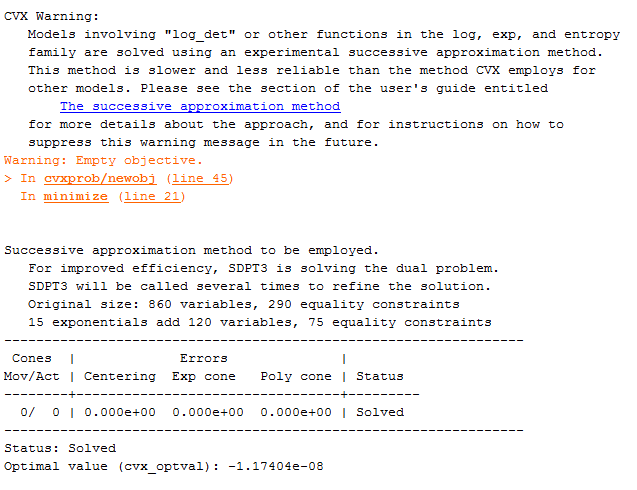# Warning: Empty objective

Hi all,

I’m trying to optimise an objective function with 5 variables, and inside CVX I wrote something like:

cvx_begin
f= some expression
minimize (f)
constraints
cvx_end

I don’t think the implementation is wrong since it was working with smaller number of variables. When I expanded the objective, I got the error “Warning: Empty objective”. Please note that, even with this warning, I’m still able to obtain
some result form CVX, but they are not meaningful.

Thank you,
S.

I think you’ll have to show us the complete program, complete without output. Do not use quiet option.

In fact, it is difficult to include the complete program since many functions are called during execution, but here a minimal example

cvx_begin
variable Q_1(n,n) hermitian semidefinite
variable Q_2(n,n) hermitian semidefinite
variable c
variable f

``````    obj_total = f( Q_1,Q_2_v ) + c_f/2 * pow_pos( norm(f - f_v, 2), 2) + c_c/2 * pow_pos( norm(c - c_v, 2), 2);

minimize( obj_total )
subject to

g( Q_1,Q_2_v ) + A * inv_pos(c) + B * inv_pos(f)  <= C;
Q_1 == hermitian_semidefinite (n); Q_2 == hermitian_semidefinite (n);
trace(Q_1) + trace(Q_2) <= D
f >= 0; c >= 0; f+c <= 1;
cvx_end
``````

where f and g are some convex functions of the indicated variables and A, B, C and D are just some constants.

Here is also a capture of one iteration during the execution of the original problemYou use f in the objective function, but haven’t shown us what it is. Perhaps there’s some 'funny business" there? I have no idea whether confusion/trouble can arise as a result of using f as both a variable and function name. Can you change it so that you don’t use f for both variable name and function?

As for the result, is it a feasible solution? The cvx_optval of -1.17404e-08 looks like it is within tolerance of being 0.

Can you show a complete minimal example of program, inputs, and output when it “works”?

Have you tried using SeDuMi? I don’t know whether the successive approximation method might be getting into trouble.

I’m quite suspicious that you’ve declared `f` as a scalar variable and you seem to be calling it as a function in `f(Q_1,Q_2_v)`. There are multiple quantities here that you haven’t defined for us, such as `f_v`, `Q_2_v`, `g`, etc. so nobody can fully appreciate what you’re doing.

I’m going to mark this one as Nonconvex, because I strongly suspect that it is, but if you provide further clarification we can pull down that tag.